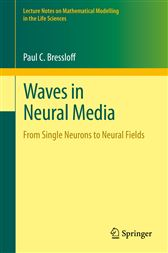# Waves in Neural Media

## Series: Lecture Notes on Mathematical Modelling in the Life SciencesAU\$149.00

Waves in Neural Media: From Single Neurons to Neural Fields surveys mathematical models of traveling waves in the brain, ranging from intracellular waves in single neurons to waves of activity in large-scale brain networks. The work provides a pedagogical account of analytical methods for finding traveling wave solutions of the variety of nonlinear differential equations that arise in such models. These include regular and singular perturbation methods, weakly nonlinear analysis, Evans functions and wave stability, homogenization theory and averaging, and stochastic processes. Also covered in the text are exact methods of solution where applicable. Historically speaking, the propagation of action potentials has inspired new mathematics, particularly with regard to the PDE theory of waves in excitable media. More recently, continuum neural field models of large-scale brain networks have generated a new set of interesting mathematical questions with regard to the solution of nonlocal integro-differential equations.

Advanced graduates, postdoctoral researchers and faculty working in mathematical biology, theoretical neuroscience, or applied nonlinear dynamics will find this book to be a valuable resource. The main prerequisites are an introductory graduate course on ordinary differential equations or partial differential equations, making this an accessible and unique contribution to the field of mathematical biology.

#### In The Press

“This is a summary of the mathematical modeling ofthe dendrites, single and multi- neuronal units. … I think this book willappeal to neuromathematicians, researchers, graduate students, fellows, andtheoretical neuroscientists.” (Joseph J. Grenier, Amazon.com, November, 2015)